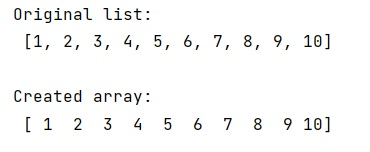# List to array conversion to use ravel() function

Learn, how to convert a list to an array using ravel() function in Python?
Submitted by Pranit Sharma, on January 09, 2023

NumPy is an abbreviated form of Numerical Python. It is used for different types of scientific operations in python. Numpy is a vast library in python which is used for almost every kind of scientific or mathematical operation. It is itself an array which is a collection of various methods and functions for processing the arrays.

## Conversion of a List to an array using ravel() method

Suppose that we are given a NumPy array we have a list in python and we want to convert it to an array to be able to use the ravel() function.

For this purpose, we will use NumPy as an array function. This function converts the input to an array. The input data is in any form that can be converted to an array. This includes lists, lists of tuples, tuples, tuples of tuples, tuples of lists, and ndarrays.
By default, the data type is inferred from the input data. It returns the Array interpretation of a. No copy is performed if the input is already a ndarray with matching dtype and order. If a is a subclass of ndarray, a base class ndarray is returned.

Let us understand with the help of an example,

## Python code for list to array conversion to use ravel() function

```# Import numpy
import numpy as np

# Creating a list
l = [1,2,3,4,5,6,7,8,9,10]

# Display original list
print("Original list:\n",l,"\n")

# Converting list into array
arr = np.asarray(l)

# Display created array
print("Created array:\n",arr,"\n")
```

Output: